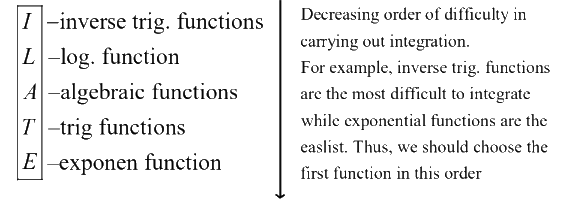# Introduction To Integration By Parts

Go back to  'Indefinite Integration'

This section will introduce you to a very powerful concept in integration. Using integration by parts, we can (theoretically) calculate the integral of the product of any two arbitrary functions. You should be very thorough with the use of this technique, since it will be extensively required in solving integration problems.

Let f(x) and g(x) be two arbitrary functions. We need to evaluate $$\int {f(x)g(x)\,\,dx}$$ . The rule for integration by parts says that :

$\fbox{{\int {f(x)g(x)\,\,dx = f(x)\int {g(x)\,\,dx - \int {\left\{ {f'(x)\int {g(x)dx} } \right\}dx} } } }}$

$\left\{ {f'(x){\text{ represents the derivative of }}f(x)} \right\}$

Translated into words (which makes it easier to remember!), this rule says that:

\fbox{\begin{align} & \text{The integral of the product of two functions = } \\ & \text{(First func) }\!\!\times\!\!\text{ (Integral of second func)-integral } \\ & \text{of }\!\!\{\!\!\text{ differential of first func }\!\!\times\!\!\text{ integral of second func }\!\!\}\!\!\text{ } \\ \end{align}}

Theoretically, we can choose any of the two functions in the product as the first function and the other as the second function. However, a little observation of the expression above will show you that since we need to deal with the integral of the second function $$\left( {\int {g(x)dx\,\,{\rm{above}}} )} \right)$$ , we should choose the second function in such a way so that it is easier to integrate; consequently, the first function should be the one that is more difficult to integrate out of the two functions. We can thus define a priority list pertaining to the choice of the first function, corresponding to the degree of difficulty in integration :The boxed letters should make it clear to you why this rule of thumb for the selection of the first function is referred to as the ILATE rule.

It is important to realise that the ILATE rule is just a guide that serves to facilitate the process of integration by parts; it is not a rule that always has to be followed; you can choose your first function contrary to the ILATE rule also if you wish to (and if you are able to integrate successfully with your choice). However, the ILATE rule works in most of the cases and is therefore widely used.

The integration by parts rule can be applied to the integral of a single function also, taking unity as the second function :

\begin{align}& \int {f(x)\,\,dx = \int {f(x) \cdot 1\,\,dx} } \\&\qquad\qquad\qquad \mathop \nearrow \limits_{{\rm{first func}}{\rm{.}}} \mathop \uparrow \limits_{{\rm{second func}}{\rm{.}}} \\&\qquad\qquad\quad= f(x)\,\,x - \int {f'(x) \cdot x\,\,dx} \end{align}

For examples,

\begin{align}& \int {\ln x\,\,dx = \int {(\ln x) \cdot 1\,\,dx} }\\ &\qquad\qquad\;= (\ln x) \cdot x - \int {\frac{1}{x} \cdot x\,\,dx} \\ &\qquad\qquad\;= (\ln x) \cdot x - \int {dx} \\& \qquad\qquad\;= x\ln x - x + C\end{align}

Before moving on to the examples, let us go through the justification of the integration by parts rule. Consider two functions $$u(x)\,\,{\rm{and }}v(x)$$ :

\begin{align}&\qquad\; \frac{d}{{dx}}(u(x) \cdot v(x)) = u(x) \cdot \frac{{d(v(x))}}{{dx}} + \frac{{d(u(x))}}{{dx}} \cdot v(x)\\\\& \Rightarrow\quad d(u(x)v(x)) = u(x)d(v(x)) + d(u(x))v(x)\\\\& \Rightarrow\quad u(x)d(v(x)) = d(u(x)v(x)) - d(u(x))v(x) \end{align}

Integration both sides, we obtain

$\int {u(x)d(v(x)) = u(x)v(x) - \int {d(u(x))v(x)} }\qquad\qquad ...\left( 1 \right)$

Let $$u(x) = f(x)$$ so that   \begin{align}d(u(x)) = f'(x)dx\end{align}   and    \begin{align}v'(x) = \frac{{d(v(x))}}{{dx}} = g(x)\end{align}  so that    $$d(v(x)) = g(x)dx$$  and  $$v(x) = \int {g(x)dx}$$ .

Substituting these values in (1), we obtain

$\int {f(x)g(x)dx = f(x)\int {g(x)dx - \int {\left( {f'(x)\int {g(x)dx} } \right)dx} } }$

This is the expression we stated earlier. Thus, this rule is simply obtainable from the product rule of differentiation.

Download SOLVED Practice Questions of Introduction To Integration By Parts for FREE
Indefinite Integration
grade 11 | Questions Set 1
Indefinite Integration
grade 11 | Answers Set 1
Indefinite Integration
grade 11 | Questions Set 2
Indefinite Integration
grade 11 | Answers Set 2